# LaTeX 数学公式总结2019年10月3日07:51:46

13200字阅读44分0秒

## LaTeX 符号总结

### 小于等于与大于等于

1. \begin{equation} \label{1_5}
2.     \begin{aligned}
3.         a \geq b & \cdots \mbox{geq, 大于等于} \\
4.         a \leq b & \cdots \mbox{leq, 小于等于}
5.     \end{aligned}
6. \end{equation}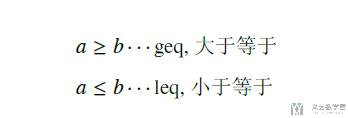### 不等号

1. \begin{equation}
2.     a \neq b
3. \end{equation}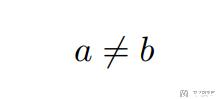### 约等于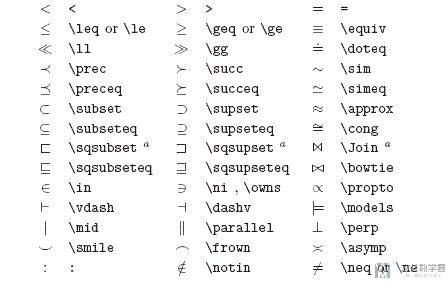### 叉乘

1. \begin{itemize}
2.     \item 叉乘, $a \times b$;
3.     \item 点乘, $a \cdot b$;
4. \end{itemize}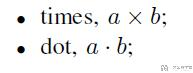### 除法

1. \begin{equation}
2.     a \div b \approx c
3. \end{equation}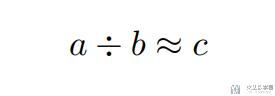### 根号的书写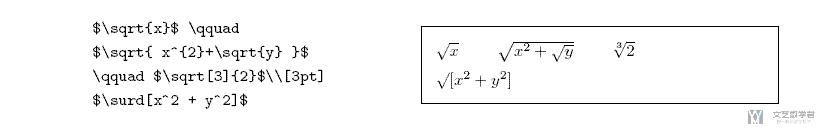### 任意和存在

1. \begin{itemize}
2.     \item $\forall s \in S$, 任意的写法;
3.     \item $\exists s \in S$, 存在的写法;
4. \end{itemize}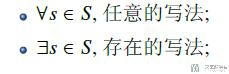### 上标撇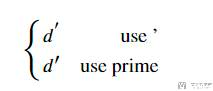### 求极限

1. \begin{equation}
2.     \lim_{n \to \infty}{(\frac{n+3}{n+2})^{n}}
3. \end{equation}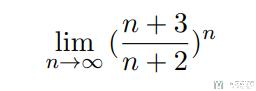### 关于微分算子的使用

1. \begin{equation}
2.     \begin{aligned}
3.     \Delta w & = -\frac{1}{2} \alpha \nabla_{w} J(w) \\
4.     & = \alpha E_{\pi}[(v_{\pi}(S) - x(S)^\top w)] \nabla_{w} (x(S)^\top w)  \\
5.     & = \alpha (v_{\pi}(S) - x(S)^\top w) x(S)
6.     \end{aligned}
7. \end{equation}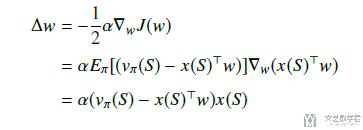### 偏导数和导数

1. \begin{equation}
2.     \begin{aligned}
3.     & = \frac{\partial{\epsilon }}{\partial{x}} \\
4.     & = \frac{\mathrm{d}y}{\mathrm{d}x_1} + \frac{\mathrm{d}y}{\mathrm{d}x_2}
5.     \end{aligned}
6. \end{equation}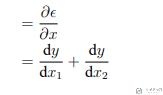### 各种括号总结

1. \begin{equation} \label{1_6}
2.     \begin{aligned}
3.         \left\{ A \right\} & \cdots \mbox{花括号} \\
4.         \left| A \right| & \cdots \mbox{单竖线} \\
5.         \left\| A \right\| & \cdots \mbox{双竖线} \\
6.         \left \langle A \right \rangle & \cdots \mbox{角括号} \\
7.         \left \lfloor A \right\rfloor & \cdots \mbox{下取证} \\
8.         \left \lceil A \right\rceil & \cdots \mbox{上取证}
9.     \end{aligned}
10. \end{equation}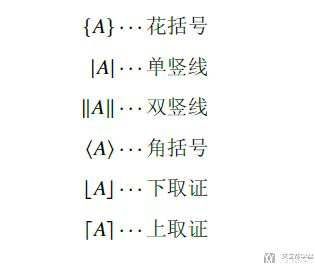### LaTeX 在公式中写入删除线

1. \usepackage{cancel} % 公式删除线

1. \begin{equation}
2.     \begin{aligned}
3.         x+\cancel{5y}=0 \\
4.         x+\bcancel{5y}=0 \\
5.         x+\xcancel{5y}=0 \\
6.         x+\cancelto{\infty}{5y}=0
7.     \end{aligned}
8. \end{equation}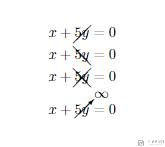1. \begin{equation}
2.     \frac{\cancel{(x+1)}(x+2)}{\cancel{(x+1)}(x+3)}
3.     =
4.     \frac{x+2}{x+3}
5. \end{equation}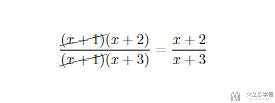### LaTeX输入交集并集等符号

1. \documentclass{article}
2. % 使用中文包
3. \usepackage[UTF8]{ctex}
4. \usepackage{algorithmic}
5. \usepackage{amsmath, amssymb,amsfonts}
6. \begin{document}
7. \begin{align}
8.     & \mathbb{R}, \mbox{实数集} \label{part1:1}\\
9.     & \mathbb{Z}, \mbox{整数集} \label{part1:2}\\
10.     & \subset, \mbox{真包含} \label{part1:3}\\
11.     & \subseteq, \mbox{包含} \label{part1:4}\\
12.     & \in, \mbox{属于} \label{part1:5}\\
13.     & \cap, \mbox{交集} \label{part1:6}\\
14.     & \cup, \mbox{并集} \label{part1:7}\\
15.     & \mid, \mbox{或} \label{part1:8}\\
16.     & \notin, \mbox{不属于} \label{part1:9}
17. \end{align}
18. \end{document}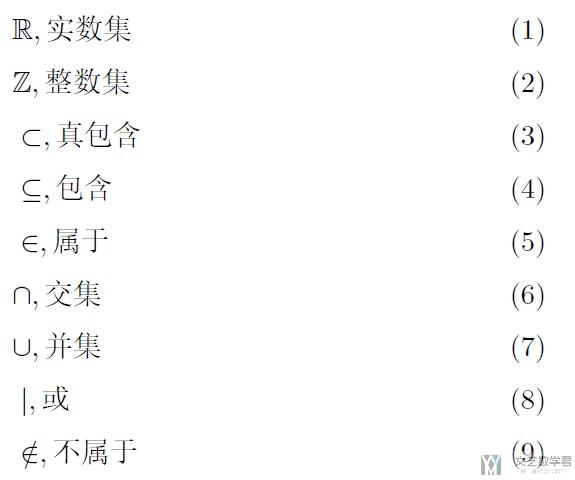### LaTeX 公式中的空格

1. \begin{align}
2.     & a \qquad b, \mbox{两个宽度} \label{part2:1}\\
3.     & a \quad b, \mbox{一个宽度} \label{part2:2}\\
4.     & a \ b, \mbox{大空格} \label{part2:3}\\
5.     & a \; b, \mbox{中等空格} \label{part2:4} \\
6.     & a \, b, \mbox{小空格} \label{part2:5} \\
7.     & a \! b, \mbox{紧贴} \label{part2:6}
8. \end{align}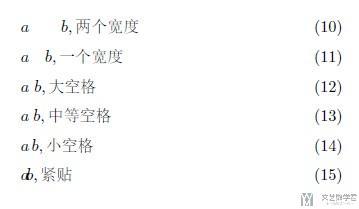### LaTeX 各种箭头符号

1. \begin{align}
2.     & \rightarrow, \mbox{右箭头} \label{part3:1}\\
3.     & \leftarrow, \mbox{左箭头} \label{part3:2}\\
4.     & \Rightarrow, \mbox{两横右箭头} \label{part3:3}\\
5.     & \Leftarrow, \mbox{两横左箭头} \label{part3:4} \\
6.     & \leftrightarrow, \mbox{双向箭头} \label{part3:5} \\
7.     & \Leftrightarrow, \mbox{双向箭头} \label{part3:6} \\
8.     & \leftrightarrows, \mbox{双向箭头} \label{part3:7} \\
9.     & \nleftarrow, \mbox{不是左箭头} \label{part3:8} \\
10.     & \nrightarrow, \mbox{不是右箭头} \label{part3:9} \\
11. \end{align}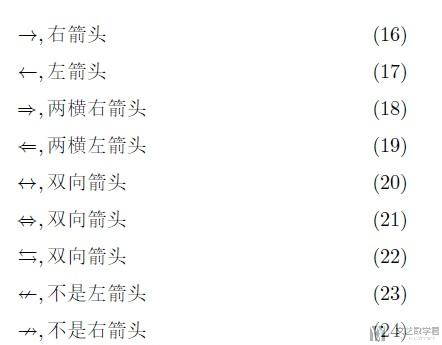### LaTeX 向量的输入

1. \vec显示结果: $\vec x$
2. \boldsymbol显示结果: $\boldsymbol x$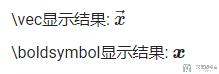### 矩阵的转置

1. \begin{equation} \label{4_8}
2.     \hat{v}(S,w) =
3.     \begin{pmatrix}
4.         1(S=s_{1})\\
5.         \vdots\\
6.         1(S=s_{n})\\
7.     \end{pmatrix}^\top
8.     \cdot
9.     \begin{pmatrix}
10.         w_{1}\\
11.         \vdots\\
12.         w_{n}\\
13.     \end{pmatrix}
14. \end{equation}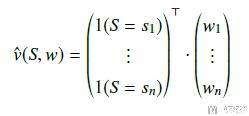### LaTeX 矩阵第一行第一列为注释

1. \begin{equation}
2.     \bordermatrix{
3.         \text{corner}&c_1&c_2&\ldots &c_n \cr
4.         r_1&a_{11} &  0  & \ldots & a_{1n} \cr
5.         r_2& 0  &  a_{22} & \ldots & a_{2n} \cr
6.         r_3& \vdots & \vdots & \ddots & \vdots \cr
7.         r_4& 0  &   0       &\ldots & a_{nn}
8.     }
9. \end{equation}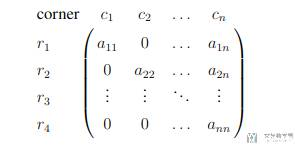### LaTeX 中矩阵输入

1. $$2. \begin{bmatrix} 3. A & B & C\\ 4. D & E & F\\ 5. G & H & I\\ 6. \end{bmatrix} 7.$$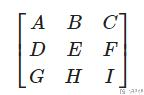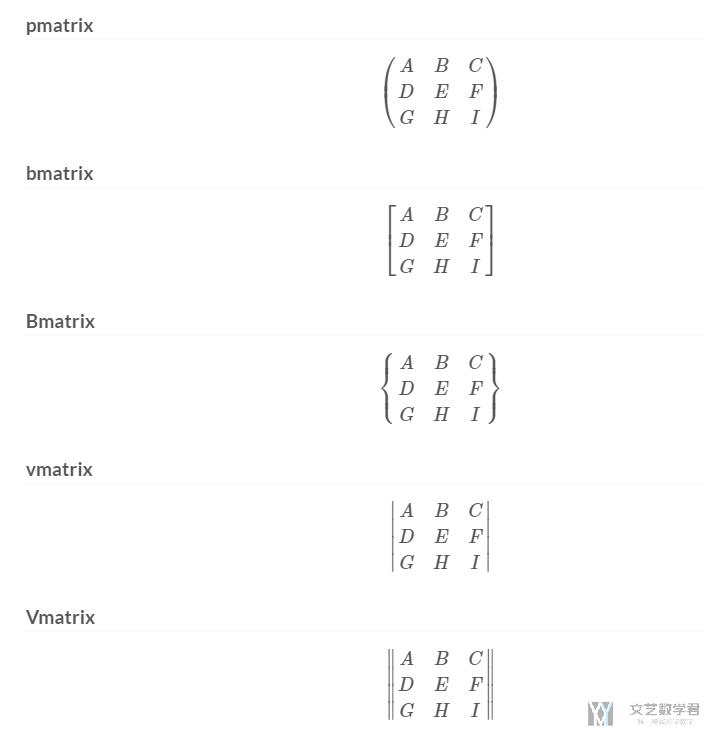### Latex的矩阵乘法

1. \begin{equation} \label{part0_9}
2.     \begin{bmatrix}
3.         v(s_{1}) \\
4.         \vdots \\
5.         v(s_{n}) \\
6.     \end{bmatrix}
7.      =
8.     \begin{bmatrix}
9.         R_{1} \\
10.         \vdots \\
11.         R_{n} \\
12.     \end{bmatrix}
13.      + \gamma \cdot
14.     \begin{bmatrix}
15.         P_{11} & \cdots & P_{1n} \\
16.         \vdots & \ddots & \vdots \\
17.         P_{n1} & \cdots & P_{nn}
18.     \end{bmatrix}
19.     \begin{bmatrix}
20.         v(s_{1}) \\
21.         \vdots \\
22.         v(s_{n}) \\
23.     \end{bmatrix}
24. \end{equation}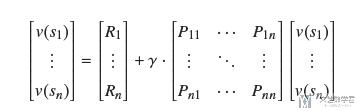### 模型中的上标和下标

1. \begin{align}
2.     & \hat{X}_{train} \\ % 顶部折线
3.     & \overline{X}_{train} \\ % 上划线
4.     & \underline{X}_{train} \\ % 下划线
5.     & \tilde{X}_{train} \\ % 顶部曲线
6. \end{align}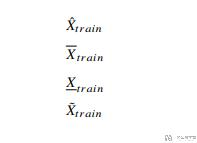### 省略号与波浪线

1. $S_{t+1} \sim p(\cdot |s_{t}, a)$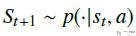1. \begin{itemize}
2.     \item 横向单个点: $\cdot$
3.     \item 横向多个点: $\cdots$
4.     \item 竖向多个点: $\vdots$
5.     \item 斜向多个点: $\ddots$
6. \end{itemize}### 关于分子分母

1. \documentclass{article}
2. % 使用中文包
3. \usepackage[UTF8]{ctex}
4. \usepackage{algorithmic}
5. \usepackage{amsmath, amssymb,amsfonts}
6. \begin{document}
7. \begin{align}
8.     & \mbox{环比增长率}: \\
9.     &&\frac{\mbox{2010年12月}}{\mbox{2010年11月}}-1=\frac{\mbox{(2010年12月-2010年11月)}}{\mbox{2010年11月}} \\
10.     & \mbox{同比增长率}: \\
11.     && \frac{\mbox{2010年12月}}{\mbox{2009年12月}}-1=\frac{\mbox{(2010年12月-2009年12月)}}{\mbox{2009年12月}}
12. \end{align}
13. \end{document}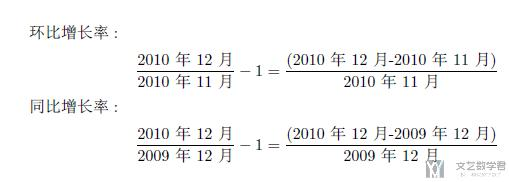### LaTeX 下方括号

1. \begin{equation}
2.     \underbrace{E_{t-2}v_t}_{=0}
3. \end{equation}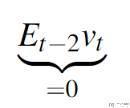1. \begin{equation}
2.     m_{i} = (
3.                 \underbrace{A, B, C}_{=1},
4.                 \underbrace{D, D, E, E, F, F}_{Group \quad Two},
5.                 \underbrace{G, H, I}_{=3},
6.             )
7. \end{equation}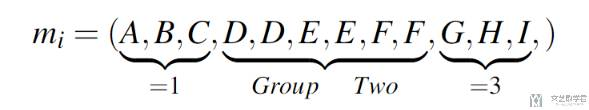### 连加符号,连乘与积分

1. Loss = -\sum_{i=1}^{N}{\hat{y_{i}}*Log(y_{i})}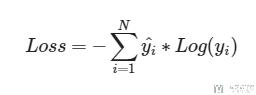1. F(x) = \prod_{i=1}^{n}{f_{i}(x)}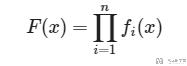1. % 微分算子
2. \newcommand*{\dif}{\mathop{}\!\mathrm{d}}
3. $\int_{-\infty}^{+\infty}{Q_{\pi}(s_{t}, a) \cdot \pi(a|s_{t})} \dif a$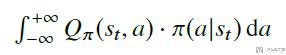### 连加符号换行

1. \begin{equation}
2.   d(\vec{x},\vec{y}) =
3.   \sum_{\substack{Z_{xy}\in\vec{Z}_{xy}\\
4.                   \forall x\in\vec{x}\\
5.                   \forall y\in\vec{y}}}
6.         f(Z_{xy})
7. \end{equation}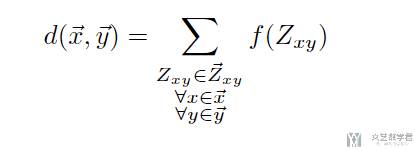### argmax 与 argmin的写法

1. \usepackage{amsmath}
2. \DeclareMathOperator*{\argmax}{arg\,max}
3. \DeclareMathOperator*{\argmin}{arg\,min}
4. a_{t}=\argmax_{a}{Q^{*}(s_{t}, a)}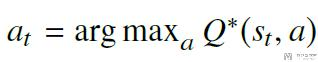## LaTeX 算法的输入

### 算法的简单例子

1. \usepackage[ruled,vlined]{algorithm2e}
2. \begin{algorithm}[H]
3.     \SetAlgoLined
4.     Initialize $Q(s, a)$, $\forall s \in S, a \in A(s)$, $Q(s,a)=0$, $N=0$ \;
5.     \While{$N<$Max Episode}{
6.         Initialize $S$ \;
7.         Choose A from S using ploicy derived Q (e.g., using $\epsilon$-greedy) \;
8.         \While{$S$ is not terminal}{
9.             Take action A, observe $R$, $S'$ \;
10.             Choose $A'$ from $S'$ using policy derived Q (e.g., using $\epsilon$-greedy) \;
11.             更新$Q(S,A)$, $Q(S,A) \leftarrow Q(S,A) + \alpha [R + \gamma Q(S',A') - Q(S,A)]$ \;
12.             $S \leftarrow S'$, $A \leftarrow A'$\;
13.         }
14.     }
15.      \caption{Sarsa算法简介}
16. \end{algorithm}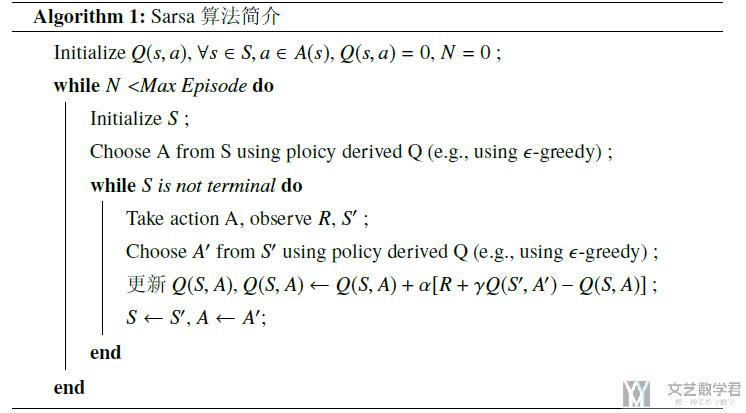### 算法加上注释

1. \usepackage[ruled]{algorithm2e} % 算法描述
2. \SetKwInput{KwInput}{Input}
3. \SetKwComment{Comment}{$\triangleright \;$}{}
4. \begin{algorithm}
5. \caption{An algorithm with caption}\label{alg:two}
6. \KwInput{batch size $B$}
7. \KwData{$n \geq 0$}
8. \KwResult{$y = x^n$}
9. $y \gets 1$\;
10. $X \gets x$\;
11. $N \gets n$\;
12. \While{$N \neq 0$}{
13.   \eIf{$N$ is even}{
14.     $X \gets X \times X$\;
15.     $N \gets \frac{N}{2}$ \Comment*[r]{This is a comment}
16.   }{\If{$N$ is odd}{
17.       $y \gets y \times X$\;
18.       $N \gets N - 1$\;
19.     }
20.   }
21. }
22. \end{algorithm}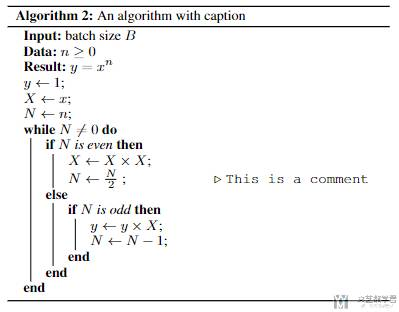### 控制算法的行间距

1. \usepackage{setspace}
2. \begin{algorithm}
3.     \setstretch{2} % 控制间距
4.     \caption{An algorithm with caption}\label{alg:two}
5.     \KwInput{batch size $B$}
6.     \KwData{$n \geq 0$}
7.     \KwResult{$y = x^n$}
8.     $y \gets 1$\;
9.     $X \gets x$\;
10.     $N \gets n$\;
11.     \While{$N \neq 0$}{
12.       \eIf{$N$ is even}{
13.         $X \gets X \times X$\;
14.         $N \gets \frac{N}{2}$ \Comment*[r]{This is a comment}
15.       }{\If{$N$ is odd}{
16.           $y \gets y \times X$\;
17.           $N \gets N - 1$\;
18.         }
19.       }
20.     }
21. \end{algorithm}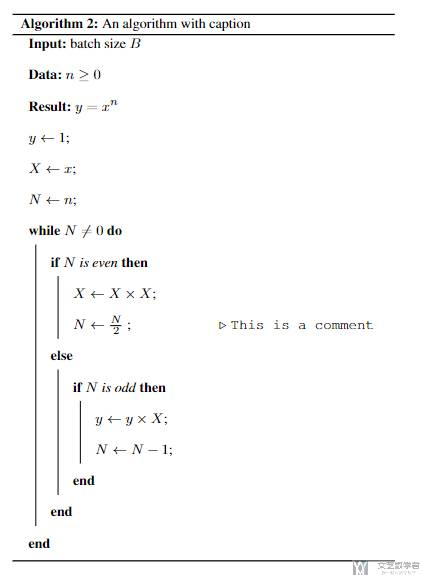## 一些其他的

### LaTeX 公式定义颜色

• 使用 color 会对剩下的所有式子上色；
• 使用 xcolor package 中的 \textcolor 可以具体对某个符号上色；

1. \begin{equation}
2.     \color{yellow} A = \textcolor{blue}{B} \textcolor{red}{-} \textcolor{green}{C}
3. \end{equation}### 公式编号的设定

1. \begin{equation}
2.     1 + 1 = 2
3. \end{equation}
4. \begin{equation} \tag{5}
5.     1 + 1 = 2
6. \end{equation}
7. \begin{equation}
8.     1 + 1 = 2
9. \end{equation}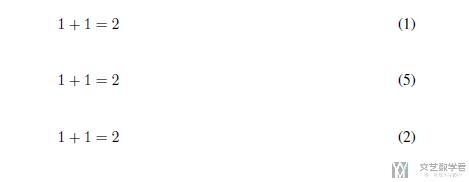### LaTeX 长公式换行并对齐

1. \begin{equation} \label{part3_shapley_eq3}
2.     \begin{aligned}
3.         f_{x}(z^{\prime}) & = f_{x}(z) + z^{\prime}, \\
4.         & for \; all \; z^{\prime} \subseteq \{x^{\prime}_1,...,x^{\prime}_n\}
5.     \end{aligned}
6. \end{equation}

1. \begin{equation} \label{part3_shapley_eq3}
2.     \begin{aligned}
3.         f_{x}(z^{\prime}) = f_{x}(z) & + z^{\prime}, \\
4.         & for \; all \; z^{\prime} \subseteq \{x^{\prime}_1,...,x^{\prime}_n\}
5.     \end{aligned}
6. \end{equation}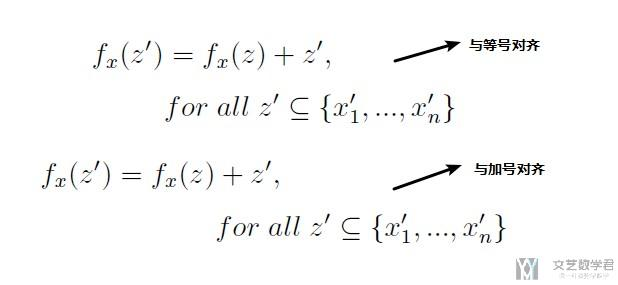### 关于大括号的使用(对齐)

1. \begin{equation} \label{part1_2}
2.     \left\{
3.     \begin{aligned}
4.         & \pi(s, UP) & = & P(A=UP|S=s) & = & 0.1 \\
5.         & \pi(s, DOWN) & = & P(A=DOWN|S=s) & = & 0.2 \\
6.         & \pi(s, LEFT) & = & P(A=LEFT|S=s) & = & 0.3 \\
7.         & \pi(s, RIGHT) & = & P(A=RIGHT|S=s) & = & 0.4 \\
8.     \end{aligned}
9.     \right.
10. \end{equation}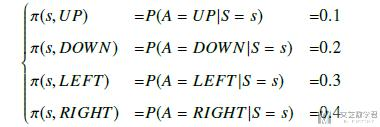### 关于多列的对齐

1. \documentclass{article}
2. \usepackage{amsmath}
3. \begin{document}
4. \begin{align*}
5. x&=y           &  w &=z              &  a&=b+c\\
6. 2x&=-y         &  3w&=\frac{1}{2}z   &  a&=b\\
7. -4 + 5x&=2+y   &  w+2&=-1+w          &  ab&=cb
8. \end{align*}
9. \end{document}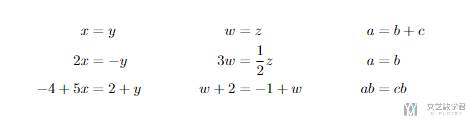1. \begin{align*}
2. x&=y           &  w &=z              &  a&=b+c\\
3. 2x&=-y         &  3w&=\frac{1}{2}z   &  a&=b\\
4. -4 + 5x&=2+y   &  w+2&=-1+w          &  ab&=cb
5. \stepcounter{equation}\tag{\theequation}\label{myeq1}\\
6. \end{align*}
7. See~\eqref{myeq1}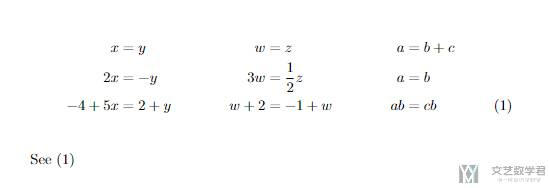### 限制 align 的宽度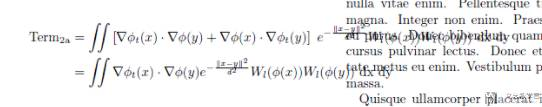1. \resizebox{.99\linewidth}{!}{
2.     \begin{minipage}{\linewidth}
3.         \begin{align*}
4.             R(2,draw,4) &= 0 &  R(2,draw,5) &= 0 & R(2,draw,done(6)) &= 0 & R(2,stop,done) &= 2 \\
5.             R(3,draw,5) &= 0 &  R(3,draw,done(6)) &= 0 & R(3,draw,done(7)) &= 0 & R(3,stop,done) &= 3 \\
6.             R(4,draw,done(6)) &= 0 &  R(4,draw,done(7)) &= 0 & R(4,draw,done(8)) &= 0 & R(4,stop,done) &= 4 \\
7.             R(5,draw,done(7)) &= 0 &  R(5,draw,done(8)) &= 0 & R(5,draw,done(9)) &= 0 & R(5,stop,done) &= 5 \\
8.             \stepcounter{equation}\tag{\theequation}\label{eq_q_2_2_2} \\
9.         \end{align*}
10.     \end{minipage}
11. }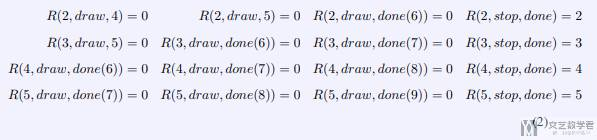### 关于多行公式后面的条件

1. \begin{equation}
2.     pow(x, n) =
3.     \left\{
4.         \begin{aligned}
5.             & pow(x, n//2)^{2} & \text{if } n \% 2 == 0 \\
6.             & pow(x, n//2)^{2} \cdot x & \text{if } n \% 2 == 1
7.         \end{aligned}
8.     \right.
9. \end{equation}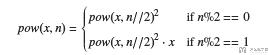### 多行公式标号

1. \documentclass{article}
2. % 使用中文包
3. \usepackage[UTF8]{ctex}
4. \usepackage{algorithmic}
5. \usepackage{amsmath, amssymb,amsfonts}
6. \begin{document}
7. \begin{align}
8.     & \mathbb{R}, \mbox{实数集} \label{part1:1}\\
9.     & \mathbb{Z}, \mbox{整数集} \label{part1:2}\\
10. \end{align}
11. \end{document}

• 微信公众号
• 关注微信公众号
•• QQ群
• 我们的QQ群号
•• 本文由 发表于 2019年10月3日07:51:46
• 转载请务必保留本文链接：https://mathpretty.com/11235.html